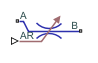# Local Restriction (IL)

Restriction in flow area in isothermal liquid network

Since R2020a

•Libraries:
Simscape / Foundation Library / Isothermal Liquid / Elements

## Description

The Local Restriction (IL) block models the pressure drop due to a localized reduction in flow area, such as a valve or an orifice, in an isothermal liquid network.

Ports A and B represent the restriction inlet and outlet. The input physical signal at port AR specifies the restriction area. Alternatively, you can specify a fixed restriction area as a block parameter.

The block icon changes depending on the value of the Restriction type parameter.

Restriction TypeBlock Icon

`Variable``Fixed`The restriction is adiabatic. It does not exchange heat with the environment.

The restriction consists of a contraction followed by a sudden expansion in flow area. The fluid accelerates during the contraction, causing the pressure to drop. In the expansion zone, if the Pressure recovery parameter is set to `off`, the momentum of the accelerated fluid is lost. If the Pressure recovery parameter is set to `on`, the sudden expansion recovers some of the momentum and allows the pressure to rise slightly after the restriction.

Local Restriction SchematicThe block equations express the mass flow rate in terms of the pressure difference between ports A and B:

`$\begin{array}{l}{\stackrel{˙}{m}}_{\text{A}}+{\stackrel{˙}{m}}_{\text{B}}=0\\ {\stackrel{˙}{m}}_{\text{A}}={C}_{d}{S}_{R}\frac{\Delta p}{{\left(\Delta {p}^{2}+\Delta {p}_{cr}^{2}\right)}^{1/4}}\sqrt{\frac{2\overline{\rho }}{P{R}_{loss}\left(1-{\left(\frac{{S}_{R}}{S}\right)}^{2}\right)}}\\ \Delta p={p}_{\text{A}}-{p}_{\text{B}}\\ \Delta {p}_{cr}=\frac{\pi }{4}\cdot \frac{\overline{\rho }}{2{S}_{R}}{\left(\frac{{\mathrm{Re}}_{cr}{\nu }_{atm}}{{C}_{d}}\right)}^{2}\end{array}$`

`$\overline{\rho }=\frac{{\rho }_{A}+{\rho }_{B}}{2}$`

where:

• Δp is the pressure differential.

• pA and pB are pressures at ports A and B, respectively.

• SR is the cross-sectional area at the restriction.

• S is the cross-sectional area at ports A and B.

• Δpcr is the critical pressure differential for the transition between the laminar and turbulent flow regimes.

• Recr is the critical Reynolds number.

• νatm is the liquid kinematic viscosity at atmospheric pressure, which is a global parameter defined by the Isothermal Liquid Properties (IL) block connected to the circuit.

• Cd is the discharge coefficient.

• $\overline{\rho }$ is the average fluid mixture density.

• ρA and ρB are fluid mixture density values at ports A and B, respectively. Equations used to compute the fluid mixture density depend on the selected isothermal liquid model. For detailed information, see Isothermal Liquid Modeling Options.

• PRloss is the pressure loss ratio.

The pressure loss ratio, PRloss, depends on the Pressure recovery parameter value:

• If pressure recovery is off, then

`$P{R}_{\text{loss}}=1.$`
• If pressure recovery is on, then

`$P{R}_{\text{loss}}=\frac{\sqrt{1-{\left(\frac{{S}_{R}}{S}\right)}^{2}\left(1-{C}_{\text{d}}^{2}\right)}-{C}_{d}\frac{{S}_{R}}{S}}{\sqrt{1-{\left(\frac{{S}_{R}}{S}\right)}^{2}\left(1-{C}_{\text{d}}^{2}\right)}+{C}_{d}\frac{{S}_{R}}{S}}.$`

The cross-sectional area at the restriction, SR, depends on the Restriction type parameter value:

• For variable restrictions,

`${S}_{\text{R}}=\left\{\begin{array}{ll}{S}_{\mathrm{min}},\hfill & AR\le {S}_{\mathrm{min}}\hfill \\ {S}_{\mathrm{max}},\hfill & AR\ge {S}_{\mathrm{max}}\hfill \\ AR,\hfill & {S}_{\mathrm{min}}`

where AR is the value of the input physical signal, and Smin and Smax are the values of the Minimum restriction area and Maximum restriction area block parameters, respectively.

• For fixed restrictions, SR is the value of the Restriction area parameter.

By default, the block assumes that the cross-sectional area at the restriction ports is much greater than the restriction area, setting the Cross-sectional area at ports A and B parameter value to `inf` and all the SR/S terms in the equations to 0, to improve computation efficiency. Specify an actual value for the Cross-sectional area at ports A and B parameter if the two cross-sectional areas are comparable in size and their ratio has an impact on flow computations.

## Ports

### Input

expand all

Input physical signal that controls the flow restriction area. The signal saturates when its value is outside the minimum and maximum restriction area limits, specified by the block parameters.

#### Dependencies

This port is visible only if you set the Restriction type parameter to `Variable`.

### Conserving

expand all

Isothermal liquid conserving port associated with the inlet or outlet of the local restriction. This block has no intrinsic directionality.

Isothermal liquid conserving port associated with the inlet or outlet of the local restriction. This block has no intrinsic directionality.

## Parameters

expand all

Select whether the restriction area can change during simulation:

• `Variable` — The input physical signal at port AR specifies the restriction area, which can vary during simulation. The Minimum restriction area and Maximum restriction area parameters specify the lower and upper bounds for the restriction area.

• `Fixed` — The restriction area, specified by the Restriction area block parameter value, remains constant during simulation. Port AR is hidden.

The lower bound for the restriction cross-sectional area. You can use this parameter to represent the leakage area. The input signal AR saturates at this value to prevent the restriction area from decreasing any further.

#### Dependencies

Enabled when the Restriction type parameter is set to `Variable`.

The upper bound for the restriction cross-sectional area. The input signal AR saturates at this value to prevent the restriction area from increasing any further.

#### Dependencies

Enabled when the Restriction type parameter is set to `Variable`.

Area normal to flow path at the restriction.

#### Dependencies

Enabled when the Restriction type parameter is set to `Fixed`.

Area normal to flow path at ports A and B. This area is assumed to be the same for the two ports. The `inf` value assumes that the cross-sectional area at the ports is much greater than the restriction area, and therefore all the SR/S terms in the block equations are negligibly small.

The discharge coefficient is a semi-empirical parameter commonly used to characterize the flow capacity of an orifice. This parameter is defined as the ratio of the actual mass flow rate through the orifice to the ideal mass flow rate.

The Reynolds number for the transition between laminar and turbulent regimes.

Specify whether to account for pressure recovery at the local restriction outlet.

## Version History

Introduced in R2020a